# Matplotlib.pyplot.stackplot() in Python

Matplotlib is a visualization library available in Python. Pyplot contains various functions that help matplotlib behave like MATLAB. It is used as matplotlib.pyplot for plotting figures, creating areas, lines, etc.

## Stackplot

Among so many functions provided by pyplot one is stackplot which will be discussed in this article. Stackplot is used to draw a stacked area plot. It displays the complete data for visualization. It shows each part stacked onto one another and how each part makes the complete figure. It displays various constituents of data and it behaves like a pie chart. It has x-label, y-label and title in which various parts can be represented by different colors.

The idea of stack plots is to show “parts to the whole” over time. It is used to represent various datasets without overlapping over each other.

Parameter Value Use
x 1-D Array It is 1 D array with N Dimensions used to give values to X-axis
y 2-D array Represents 2 D array of M*N Dimension which is unstacked.
Colors Contains List or tuple of Colors It is used to give range of colors to represent data with default value is None.
Baseline {‘zero’, ‘sym’, ‘wiggle’, ‘weighted_wiggle’} Zero means constant baseline.
Sym which is symmetric around zero value.
wiggle it will minimize value of the sum of squares.
**kwargs List of other keywords Other Arguments or keywords.

Syntax:

matplotlib.pyplot.stackplot(x, *args, labels=(), colors=None, baseline=’zero’, data=None, **kwargs)

Example #1 : Using Stackplot
The code describes the x-axis as number of days from Moday to Friday while Y-axis is represented by No of Study and playing time represented by red and cyan color respectively.

 `import` `matplotlib.pyplot as plt ` ` `  `# List of Days ` `days ``=` `[``1``, ``2``, ``3``, ``4``, ``5``] ` ` `  `# No of Study Hours ` `Studying ``=` `[``7``, ``8``, ``6``, ``11``, ``7``] ` ` `  `# No of Playing Hours ` `playing ``=`  `[``8``, ``5``, ``7``, ``8``, ``13``] ` ` `  `# Stackplot with X, Y, colors value ` `plt.stackplot(days, Studying, playing, ` `              ``colors ``=``[``'r'``, ``'c'``]) ` ` `  `# Days ` `plt.xlabel(``'Days'``) ` ` `  `# No of hours ` `plt.ylabel(``'No of Hours'``) ` ` `  `# Title of Graph ` `plt.title('Representation of Study ``and` `\ ` `Playing wrt to Days') ` ` `  `# Displaying Graph ` `plt.show() `

Output: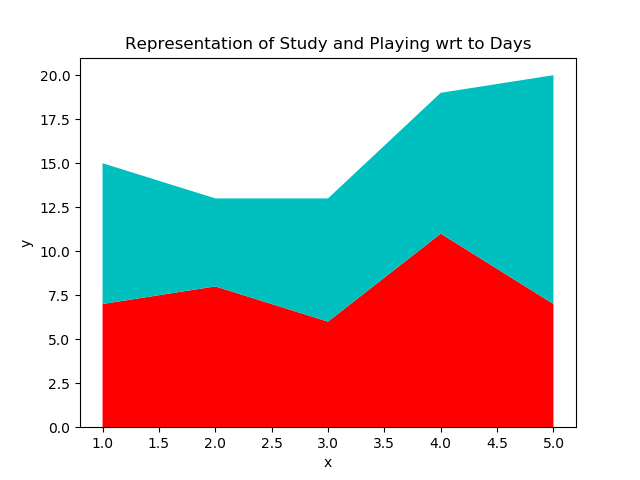Example #2 : Using Stackplot

 `import` `matplotlib.pyplot as plt ` ` `  `# List of 7-days ` `days ``=` `[x ``for` `x ``in` `range``(``0``, ``7``)] ` ` `  `# List of Suspected cases ` `Suspected ``=` `[``12``, ``18``, ``35``, ``50``, ``72``, ``90``, ``100``] ` ` `  `# List of Cured Cases ` `Cured ``=` `[``4``, ``8``, ``15``, ``22``, ``41``, ``55``, ``62``] ` ` `  `# List of Number of deaths ` `Deaths ``=` `[``1``, ``3``, ``5``, ``7``, ``9``, ``11``, ``13``] ` ` `  `# Plot x-labels, y-label and data ` `plt.plot([], [], color ``=``'blue'``,  ` `         ``label ``=``'Suspected'``) ` `plt.plot([], [], color ``=``'orange'``, ` `         ``label ``=``'Cured'``) ` `plt.plot([], [], color ``=``'brown'``, ` `         ``label ``=``'Deaths'``) ` ` `  `# Implementing stackplot on data ` `plt.stackplot(days, Suspected, Cured,  ` `              ``Deaths, baseline ``=``'zero'``,  ` `              ``colors ``=``[``'blue'``, ``'orange'``,  ` `                       ``'brown'``]) ` ` `  `plt.legend() ` ` `  `plt.title(``'No of Cases'``) ` `plt.xlabel(``'Day of the week'``) ` `plt.ylabel(``'Overall cases'``) ` ` `  `plt.show() `

Output:

Below represents the output of graph if the value of baseline is set to zero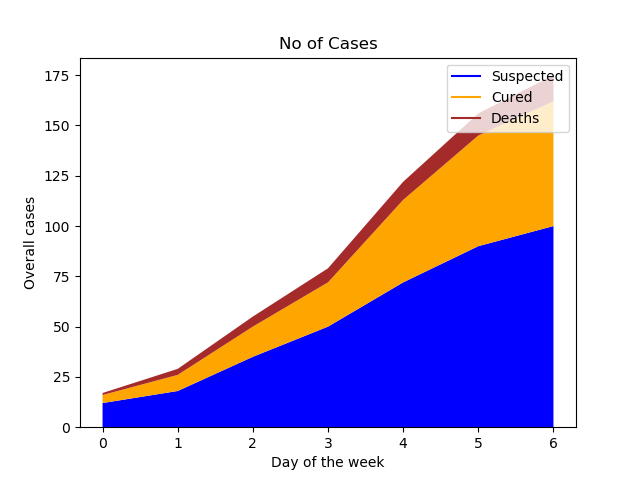Below represents the output of graph if the value of baseline is set to sym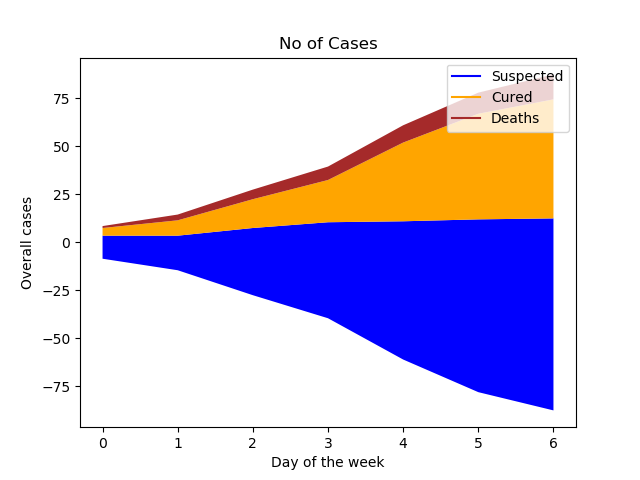Below represents the output of graph if the value of baseline is set to wiggle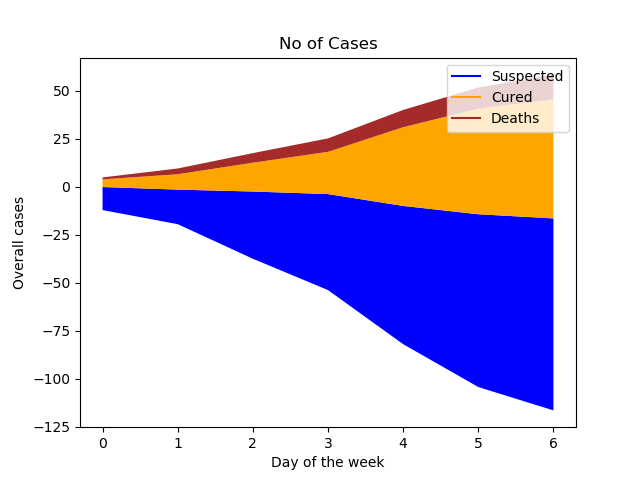Below represents the output of graph if the value of baseline is set to weighted_wiggle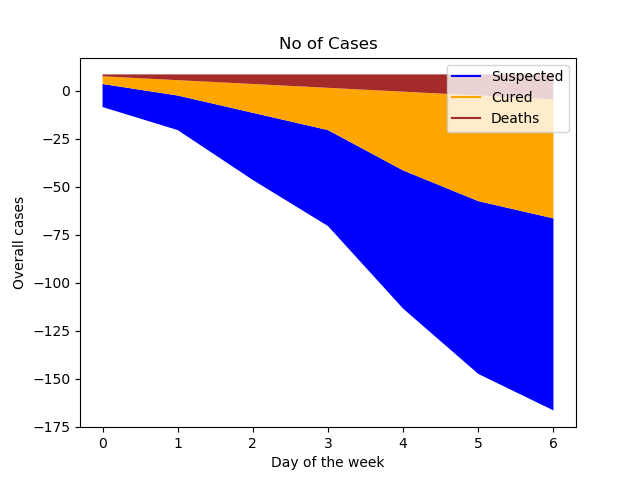My Personal Notes arrow_drop_upCheck out this Author's contributed articles.

If you like GeeksforGeeks and would like to contribute, you can also write an article using contribute.geeksforgeeks.org or mail your article to contribute@geeksforgeeks.org. See your article appearing on the GeeksforGeeks main page and help other Geeks.

Please Improve this article if you find anything incorrect by clicking on the "Improve Article" button below.

Article Tags :

Be the First to upvote.

Please write to us at contribute@geeksforgeeks.org to report any issue with the above content.